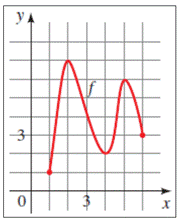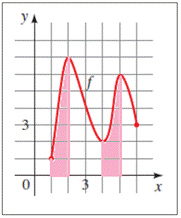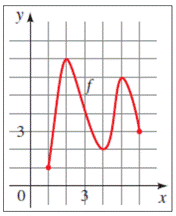# To fill in the gaps : with reference to the provided graph### Precalculus: Mathematics for Calcu...

6th Edition
Stewart + 5 others
Publisher: Cengage Learning
ISBN: 9780840068071### Precalculus: Mathematics for Calcu...

6th Edition
Stewart + 5 others
Publisher: Cengage Learning
ISBN: 9780840068071

#### Solutions

Chapter 2.3, Problem 3E

a.

To determine

## To fill in the gaps: with reference to the provided graph

Expert Solution

If f is increasing on an interval, then the y -values of the points on the graph increase as the x -values increase. From the graph of f , we see that f is increasing on the interval [1, 2] and [4, 5] .

### Explanation of Solution

Given information: if f is increasing on an interval, then the y -values of the points on the graph____ as the x -values increase. From the graph of f , we see that f is increasing on the interval _____ and _____ .From the provided information, consider,As f increases the height if the function f ( x ) also increases, thus, y-values of the points on the graph increase as the x-values increase. In the graph provided, f is increasing on the intervals [1, 2] and [4, 5].

Hence, if f is increasing on an interval, then the y -values of the points on the graph increase as the x -values increase. From the graph of f , we see that f is increasing on the interval [1, 2] and [4, 5] .

b.

To determine

### To fill in the gaps: with reference to the provided graph

Expert Solution

If f is decreasing on an interval, then the y -values of the points on the graph decrease as the x -values increase. From the graph of f , we see that f is increasing on the interval [2, 4] and [5, 6] .

### Explanation of Solution

Given information: If f is decreasing on an interval, then the y- values of the points on the graph ______ as the x -values increases. From the graph, we see that f is decreasing on the interval ____ and ____.From the provided information, consider,As f decreases, the height of the function f ( x ) also decreases, thus, y-values of the points on the graph decrease as the x-values increase. In the graph provided, f is increasing on the intervals [2, 3] and [5, 6].

Hence, if f is decreasing on an interval, then the y -values of the points on the graph decrease as the x -values increase. From the graph of f , we see that f is increasing on the interval [2, 4] and [5, 6] .

### Have a homework question?

Subscribe to bartleby learn! Ask subject matter experts 30 homework questions each month. Plus, you’ll have access to millions of step-by-step textbook answers!